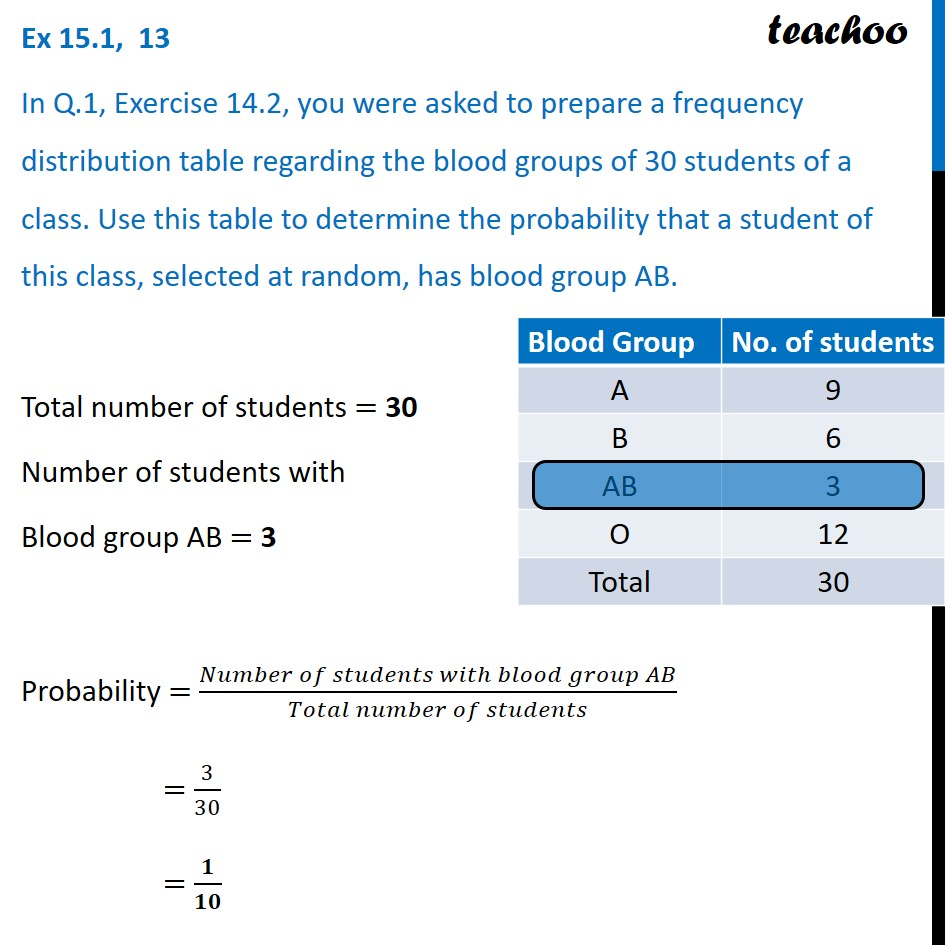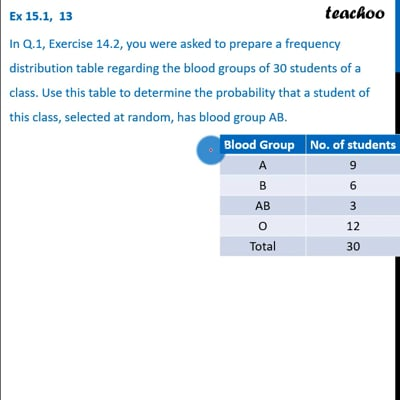Questions - Basic Probability

Probability
Serial order wise
• Questions - Basic Probability

• ExamplesThis video is only available for Teachoo black users

Learn in your speed, with individual attention - Teachoo Maths 1-on-1 Class

### Transcript

Question 13 In Q.1, Exercise 14.2, you were asked to prepare a frequency distribution table regarding the blood groups of 30 students of a class. Use this table to determine the probability that a student of this class, selected at random, has blood group AB. Total number of students = 30 Number of students with Blood group AB = 3 Probability = (𝑁𝑢𝑚𝑏𝑒𝑟 𝑜𝑓 𝑠𝑡𝑢𝑑𝑒𝑛𝑡𝑠 𝑤𝑖𝑡ℎ 𝑏𝑙𝑜𝑜𝑑 𝑔𝑟𝑜𝑢𝑝 𝐴𝐵)/(𝑇𝑜𝑡𝑎𝑙 𝑛𝑢𝑚𝑏𝑒𝑟 𝑜𝑓 𝑠𝑡𝑢𝑑𝑒𝑛𝑡𝑠) = 3/30 = 𝟏/𝟏𝟎